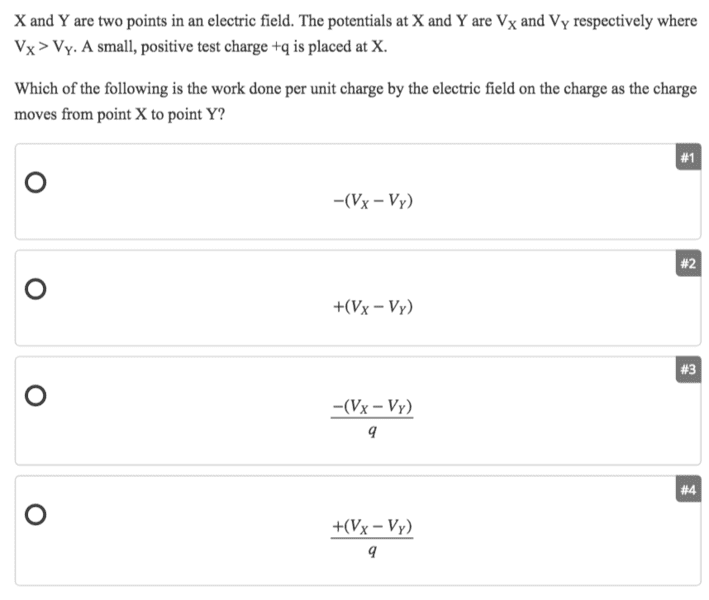# Work done per unit charge by electric field on charge

## Homework Statement## The Attempt at a Solution

I know the units for V is J/C so #3 and #4 are wrong. But how do I determine the signs?

#### Attachments

kuruman
Homework Helper
Gold Member
2021 Award
Is the work done by the electric field positive or negative? What do you need to ascertain in order to answer this question? Hint: When is the work done by a force positive and when is it negative?

Is the work done by the electric field positive or negative? What do you need to ascertain in order to answer this question? Hint: When is the work done by a force positive and when is it negative?
Work is positive when force is in same direction as displacement. So here the direction is X to Y and the force is also from X to Y because Vx>Vy?

kuruman